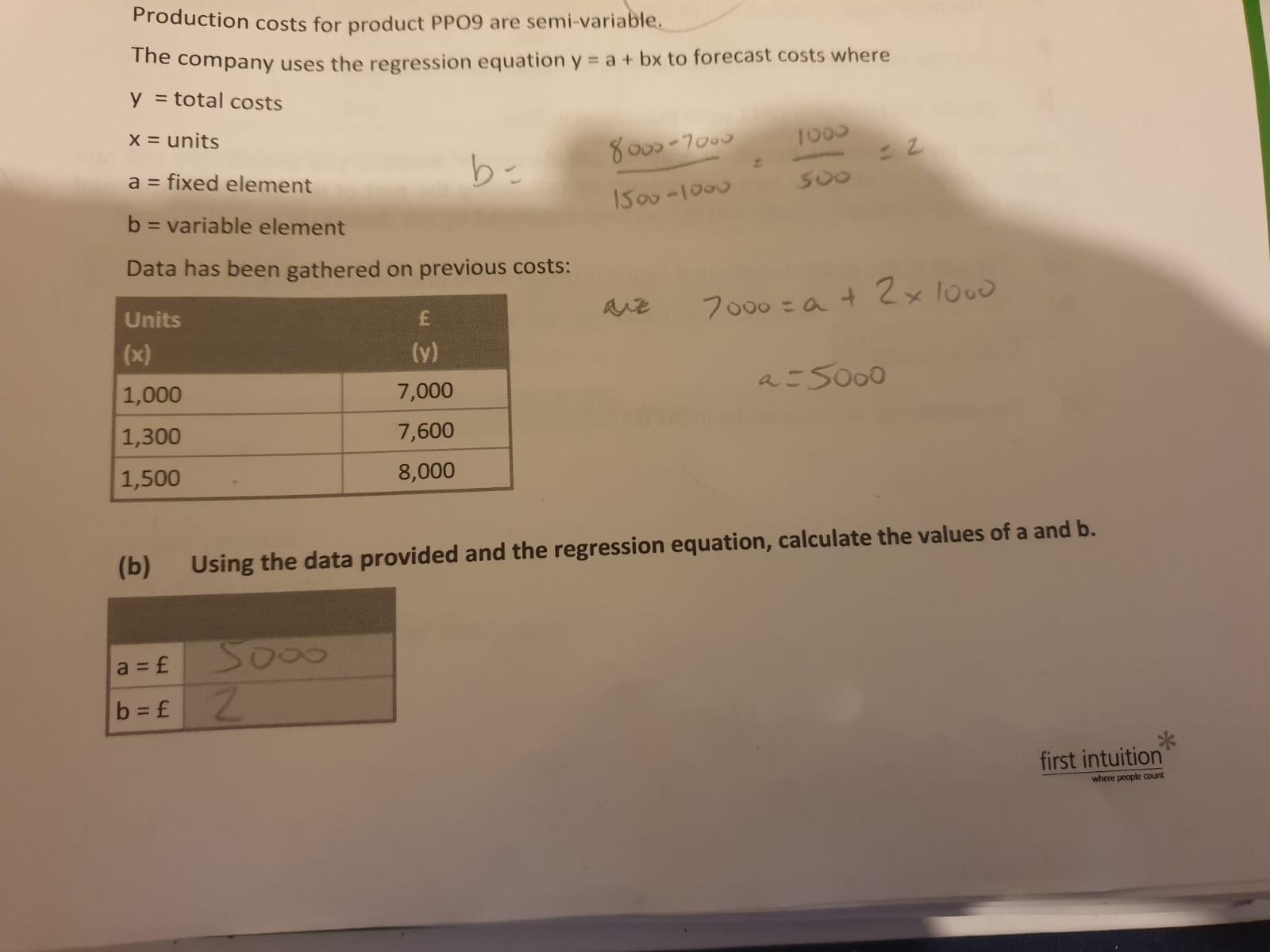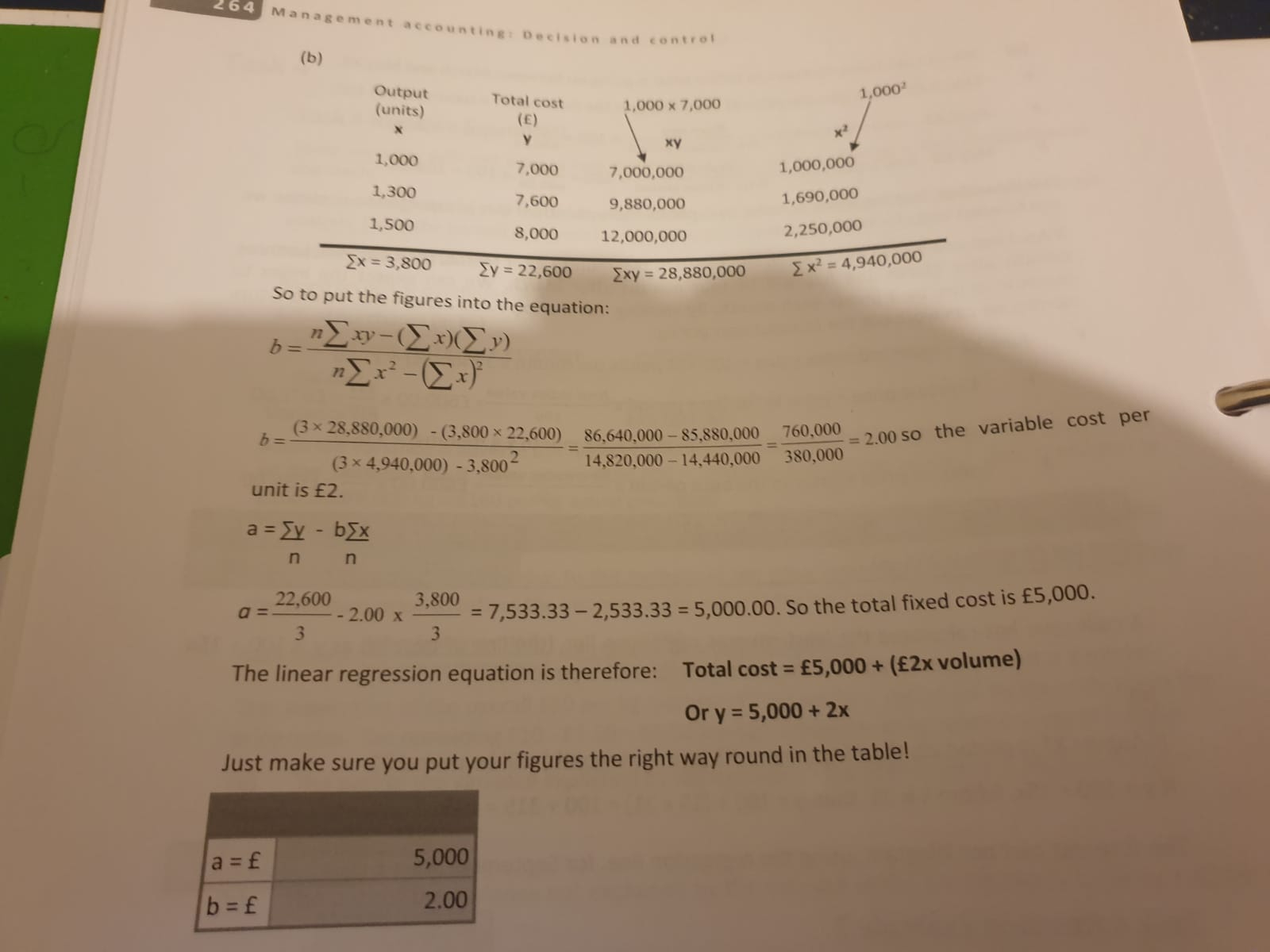# Regression Equation .....again

Hi,

Just a quick question.

I'm going through some practice questions in the first intuition questions banks.

I can get the correct answer to the following question using the high - low method of calculating value of b....Pretty straightforward and very good and all.....but the answers say the correct way of finding the same answer is the far more convoluted method of ∑x ∑y ∑xy ∑x 2 (squared)My question I guess is do we ever need to use the longwinded ∑x ∑y....etc etc method? and in what circumstance is it needed rather than the much easier high low method?

My exam is tomorrow so doing lots of last day revision.Thanks

## Comments

• Registered, Tutor Posts: 196
I'm afraid I don't understand why First Intuition use the method of least squares. The AAT's specification for this module states that:

Students for semi-variable costs need to be able to:
• use the high-low method to extract the fixed and variable elements, including making
adjustments for a step up in cost or a quantity discount.

Calculate key statistical indicators
Students need to be able to:
• calculate
- index numbers
- Time series analysis – moving averages, seasonal variations and trend information
• use the regression equation (e.g. y= a+bx)
• calculate the outputs from various statistical calculations.

(no mention of "least squares" here!)

We know that the high-low method is incredibly approximate, but you are very unlikely to get a precise result from just 3 data points. Furthermore, this is management accounting; the results simply need to be good enough to make a rational decision.
• Thanks again Peter.

that's good to know. I mean I can do the least squares method, but it's time consuming and more likely to make mistakes for the same answer.

Was it ever needed? ie pre aq2016? maybe they just haven't updated the notes properly for AQ2016. It's a bit annoying, especially considering what I've paid them for lessons and their material......
• Registered, Tutor Posts: 196
AAT has certainly used high-low ever since they started offering nvqs, which (I think) was 2003.
Sign In or Register to comment.